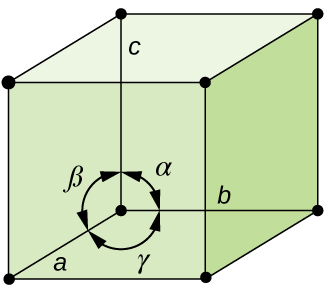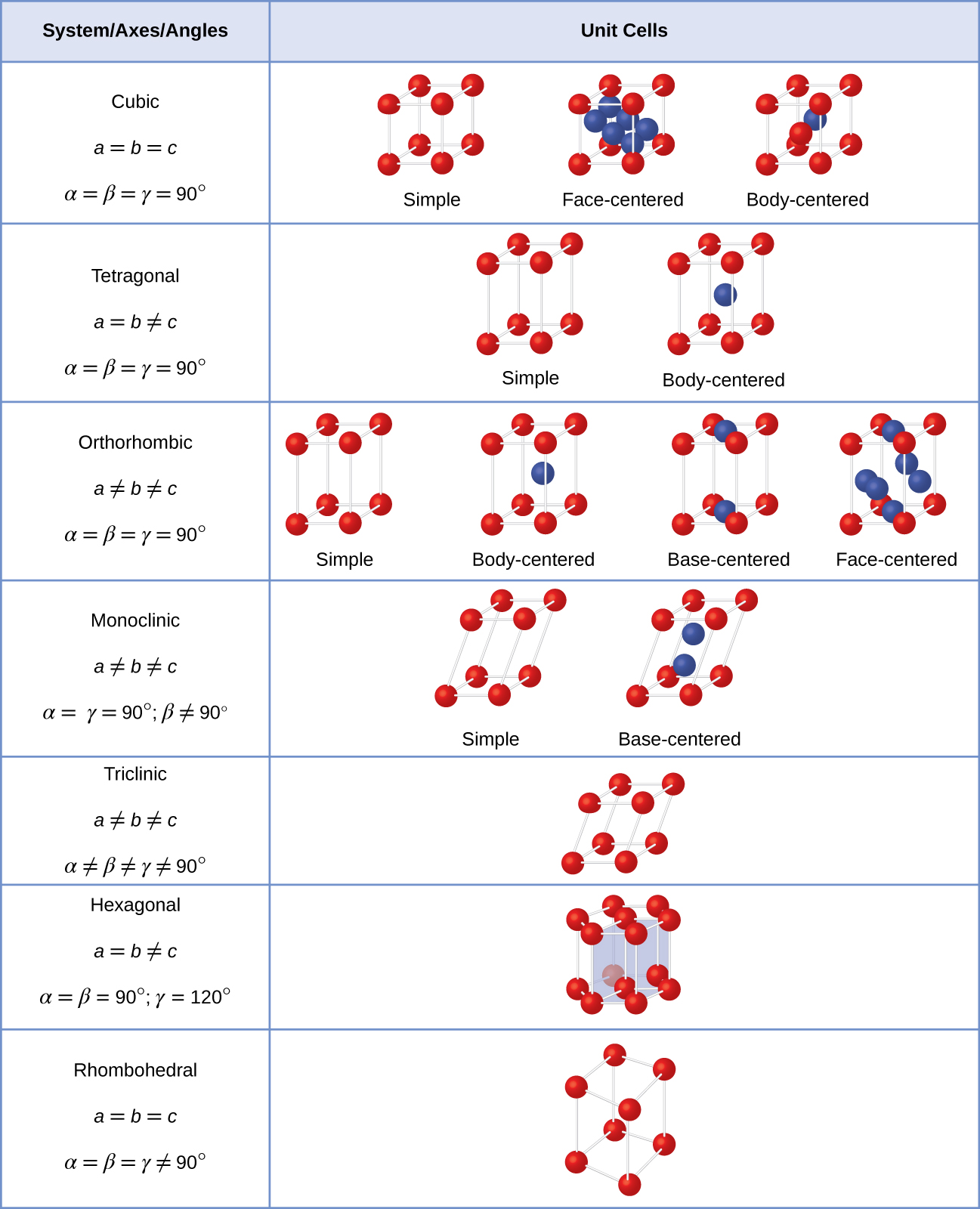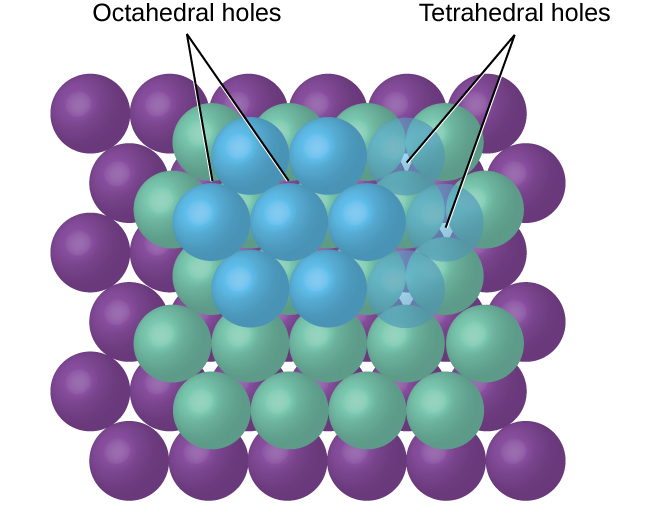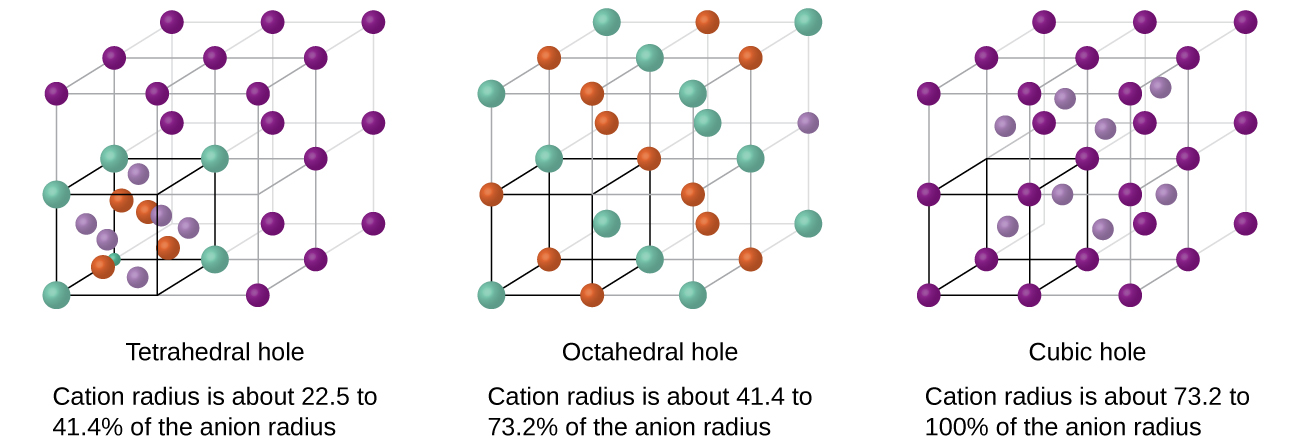# 10.6 Lattice structures in crystalline solids  (Page 5/29)

 Page 5 / 29

In general, a unit cell is defined by the lengths of three axes ( a , b , and c ) and the angles ( α , β , and γ ) between them, as illustrated in [link] . The axes are defined as being the lengths between points in the space lattice. Consequently, unit cell axes join points with identical environments.A unit cell is defined by the lengths of its three axes ( a , b , and c ) and the angles ( α , β , and γ ) between the axes.

There are seven different lattice systems, some of which have more than one type of lattice, for a total of fourteen different unit cells, which have the shapes shown in [link] .There are seven different lattice systems and 14 different unit cells.

## The structures of ionic crystals

Ionic crystals consist of two or more different kinds of ions that usually have different sizes. The packing of these ions into a crystal structure is more complex than the packing of metal atoms that are the same size.

Most monatomic ions behave as charged spheres, and their attraction for ions of opposite charge is the same in every direction. Consequently, stable structures for ionic compounds result (1) when ions of one charge are surrounded by as many ions as possible of the opposite charge and (2) when the cations and anions are in contact with each other. Structures are determined by two principal factors: the relative sizes of the ions and the ratio of the numbers of positive and negative ions in the compound.

In simple ionic structures, we usually find the anions, which are normally larger than the cations, arranged in a closest-packed array. (As seen previously, additional electrons attracted to the same nucleus make anions larger and fewer electrons attracted to the same nucleus make cations smaller when compared to the atoms from which they are formed.) The smaller cations commonly occupy one of two types of holes (or interstices) remaining between the anions. The smaller of the holes is found between three anions in one plane and one anion in an adjacent plane. The four anions surrounding this hole are arranged at the corners of a tetrahedron, so the hole is called a tetrahedral hole    . The larger type of hole is found at the center of six anions (three in one layer and three in an adjacent layer) located at the corners of an octahedron; this is called an octahedral hole    . [link] illustrates both of these types of holes.Cations may occupy two types of holes between anions: octahedral holes or tetrahedral holes.

Depending on the relative sizes of the cations and anions, the cations of an ionic compound may occupy tetrahedral or octahedral holes, as illustrated in [link] . Relatively small cations occupy tetrahedral holes, and larger cations occupy octahedral holes. If the cations are too large to fit into the octahedral holes, the anions may adopt a more open structure, such as a simple cubic array. The larger cations can then occupy the larger cubic holes made possible by the more open spacing.A cation’s size and the shape of the hole occupied by the compound are directly related.

There are two tetrahedral holes for each anion in either an HCP or CCP array of anions. A compound that crystallizes in a closest-packed array of anions with cations in the tetrahedral holes can have a maximum cation:anion ratio of 2:1; all of the tetrahedral holes are filled at this ratio. Examples include Li 2 O, Na 2 O, Li 2 S, and Na 2 S. Compounds with a ratio of less than 2:1 may also crystallize in a closest-packed array of anions with cations in the tetrahedral holes, if the ionic sizes fit. In these compounds, however, some of the tetrahedral holes remain vacant.

what are oxidation numbers
pls what is electrolysis
Electrolysis is the process by which ionic substances are decomposed (broken down) into simpler substances when an electric current is passed through them. ... Electricity is the flow of electrons or ions. For electrolysis to work, the compound must contain ions.
AZEEZ
thanks
Idowu
what is the basicity of an atom
basicity is the number of replaceable Hydrogen atoms in a Molecule. in H2SO4, the basicity is 2. in Hcl, the basicity is 1
Inemesit
how to solve oxidation number
mention some examples of ester
do you mean ether?
Megan
what do converging lines on a mass Spectra represent
would I do to help me know this topic ?
Bulus
oi
Amargo
what the physic?
who is albert heistein?
Bassidi
similarities between elements in the same group and period
what is the ratio of hydrogen to oxulygen in carbohydrates
bunubyyvyhinuvgtvbjnjnygtcrc
yvcrzezalakhhehuzhbshsunakakoaak
what is poh and ph
please what is the chemical configuration of sodium
Sharon
2.8.1
david
1s²2s²2p⁶3s¹
Haile
2, 6, 2, 1
Salman
1s2, 2s2, 2px2, 2py2, 2pz2, 3s1
Justice
1s2,2s2,2py2,2
Maryify
1s2,2s2,2p6,
Francis
1s2,2s2,2px2,2py2,2pz2,3s1
Nnyila
what is criteria purity
cathode is a negative ion why is it that u said is negative
cathode is a negative electrode while cation is a positive ion. cation move towards cathode plate.
king
CH3COOH +NaOH ,complete the equation
compare and contrast the electrical conductivity of HCl and CH3cooH
The must be in dissolved in water (aqueous). Electrical conductivity is measured in Siemens (s). HCl (aq) has higher conductivity, as it fully ionises (small portion of CH3COOH (aq) ionises) when dissolved in water. Thus, more free ions to carry charge.
Abdelkarim
HCl being an strong acid will fully ionize in water thus producing more mobile ions for electrical conduction than the carboxylic acid
Valentine
differiante between a weak and a strong acid
david
how can I tell when an acid is weak or Strong
Amarachi
an aqueous solution of copper sulphate was electrolysed between graphite electrodes. state what was observed at the cathode
write the equation for the reaction that took place at the anode
Bakanya
what is enthalpy of combustion
Bakanya
Enthalpy change of combustion: It is the enthalpy change when 1 mole of substance is combusted with excess oxygen under standard conditions. Elements are in their standard states. Conditions: pressure = 1 atm Temperature =25°C
Abdelkarim
Observation at Cathode: Cu metal deposit (pink/red solid).
Abdelkarim
Equation at Anode: (SO4)^2- + 4H^+ + 2e^- __> SO2 + 2H2O
Abdelkarim
Equation : CuSO4 -> Cu^2+ + SO4^2- equation at katode: 2Cu^2+ + 4e -> 2Cu equation at anode: 2H2O -> 4H+ + O2 +4e at the anode which reacts is water because SO4 ^ 2- cannot be electrolyzed in the anode
NikenByByBy Robert MurphyBy OpenStaxBy Jazzycazz JacksonBy Keyaira BraxtonBy RhodesBy Mistry BhaveshBy Sarah WarrenBy OpenStaxBy Inderjeet BrarBy Cath Yu